www.batmath.it

Examples of linear systems

1 - Solve the system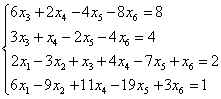.

The augmented matrix A|b of the system is: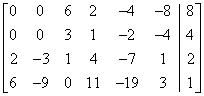,

which is row equivalent to the reduced row-echelon matrix B|c: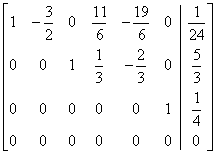.

The solution of the system is easily found: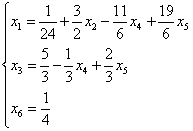. The other unknowns are completely arbitrary. This system has n=6, m=4, r=3, and so ∞6-3=∞3 solutions.

2 - Solve the system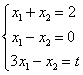, where t is a real number.

The augmented matrix and its reduced form are: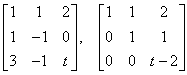. The system is consistent if and only if t=2. In this case the reduced row-echelon form of the matrix is: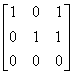. We read off the unique solution of the system: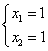. From a geometrical point of view this result can be interpreted as follows: given two non parallel straight lines and a third variable straight line, find the value of t for which this third line has a unique point in common with the preceding two.

3 - Solve the system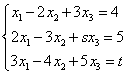, where s and t are real numbers.

Write the augmented matrix A|b: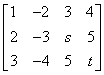. Now start applying Gauss-Jordan algorithm.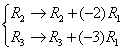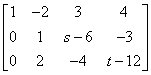;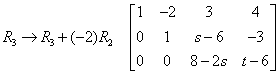.

Now we must distinguish between to cases:

• If s4 the system has only one solution that we found proceding from bottom to top: we begin finding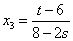, then we find the second unknown using the second equation and finally the first unknown using the first equation. There is no need to produce the reduced row-echelon form of the matrix.
• If s=4 we must check the value of t.
• If t6 the system is inconsistent.
• If t=6 we produce the following matrix: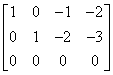. We can easily read off the solution: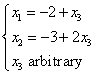.
first published on march 15 2002 - last updated on september 01 2003